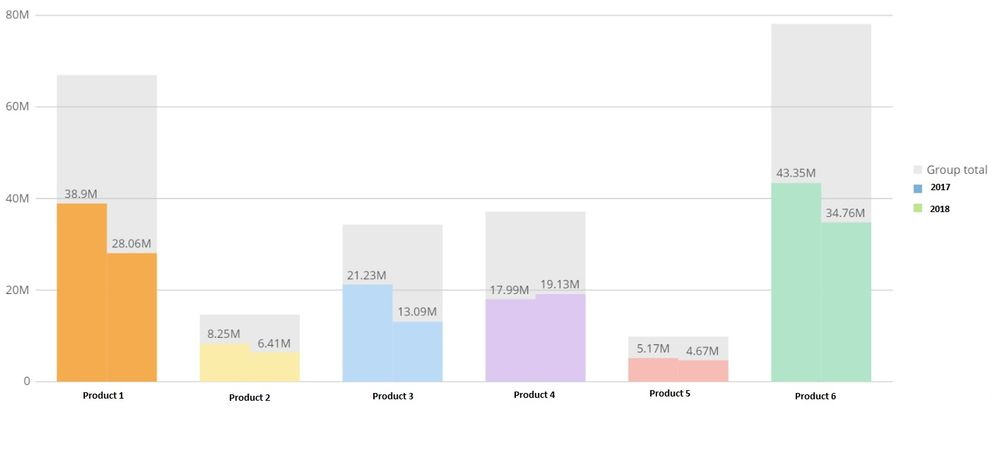# YoY Percentage Delta Calculation in Nested Bar Charts

Hello,

Is there a way to calculate on the fly the percentage delta within a nested bar chart? Instead of displaying year's totals, I would like to disply the perscentage deltas in the graph below.

Any ideas?Thanks!

• You can do a beast mode calculation finding the delta between the two years and use that as your Y value. You can than change the format of the number to display as a percentage. Let me know if this works for you!

• I have tried two of the examples online (c-p/p), but not joy:

(SUM(CASE WHEN YEAR(`CloseDate`) = YEAR('NOW') THEN `OpportunityAmount__c` ELSE 0 END)-SUM(CASE WHEN YEAR(`CloseDate`)= YEAR(DATE_SUB('NOW', INTERVAL 1 YEAR))AND month(`CloseDate`) <= month('NOW') THEN `OpportunityAmount__c` ELSE 0 END)) / SUM(CASE WHEN YEAR(`CloseDate`)= YEAR(DATE_SUB('NOW', INTERVAL 1 YEAR)) AND month(`CloseDate`) <= month('NOW') THEN `OpportunityAmount__c` ELSE 0 END)

• I believe there is not really a way to do what I want to do using this type of graph.

Agree?